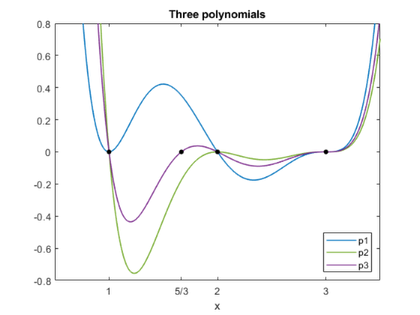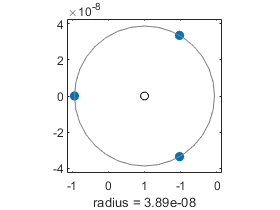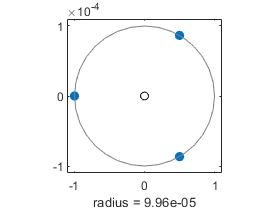Pejorative Manifolds of Polynomials and Matrices, part 1

In an unpublished 1972 technical report "Conserving confluence curbs ill-condition," Velvel Kahan coined the descriptive term pejorative manifold. In case you don't use it in everyday conversation, pejorative means "expressing contempt or disapproval."

Velvel's report concerns polynomials with multiple roots, which are usually regarded with contempt because they are so badly conditioned. But Velvel's key observation is that although multiple roots are sensitive to arbitrary perturbation, they are insensitive to perburbations that preserve multiplicity. The same observation can be applied to matrix inverses and eigenvalues.

Contents

The manifold

In this example, the pejorative manifold $\mathcal{M}$ is the set of all sixth degree polynomials which have a zero of multiplicity three at x = 3. These are severe restrictions, of course, and $\mathcal{M}$ is a tiny subset of the set of all polynomials. But if we stay within $\mathcal{M}$, life is not nearly so harsh.

Two polynomials

First a pair of polynomials with multiple roots. All of our polynomials have a root of multplicity three at x = 3 as well as other multiple roots. This one a double root at x = 1 and a simple root at x = 2.

x = sym('x');

p1 = (x-3)^3*(x-2)*(x-1)^2;

$$p1 = {\left(x-1\right)}^2\,\left(x-2\right)\,{\left(x-3\right)}^3$$

The next one has a double root at x = 2 and a simple root at x = 1.

p2 = (x-3)^3*(x-2)^2*(x-1);

$$p2 = \left(x-1\right)\,{\left(x-2\right)}^2\,{\left(x-3\right)}^3$$

To summarize, we have two polynomials, p1 and p2 . Both have degree six and have a triple root at x = 3. In addition, pk has a double root at x = k .

Confirm

Let's see the coefficients.

p1 = expand(p1);

$$p1 = x^6-13\,x^5+68\,x^4-182\,x^3+261\,x^2-189\,x+54$$

p2 = expand(p2);

$$p2 = x^6-14\,x^5+80\,x^4-238\,x^3+387\,x^2-324\,x+108$$

We can solve them exactly and verify that they have the desired roots.

z1 = solve(p1)'
z2 = solve(p2)'
z1 =
[ 1, 1, 2, 3, 3, 3]
z2 =
[ 1, 2, 2, 3, 3, 3]

Convex combination

Now let's take a convex linear combination. I am using 1/3 and 2/3, but I could use any other weights as long as their sum is 1. Any such convex linear combination is still in $\mathcal{M}$.

p3 = 1/3*p1 + 2/3*p2;

$$p3 = x^6-\frac{41\,x^5}{3}+76\,x^4-\frac{658\,x^3}{3}+345\,x^2-279\,x+90$$

This still has the triple root at x = 3. The roots at x = 1 and x = 2 are now simple and the root at x = 5/3 is the convex linear combination of 1 and 2 with the same coefficents, 5/3 = 1/3*1 + 2/3*2.

z = solve(p3)'
z =
[ 1, 5/3, 2, 3, 3, 3]

Looking at plots of the three polynomials, you can appreciate how the triple root at 3 is more sensitive than the blue double root at 1 or the green double root at 2, which are, in turn, more sensitive and any of the simple roots.

plot_polys(p1,p2,p3)Floating point haze

Now a small perburbation. Take a convex linear combination with an irrational weight and use vpa, the variable precision floating point arithmetic. We are in a floating point haze with thickness $10^{-32}$ surrounding $\mathcal{M}$.

digits(32)
w = 2/(1+sqrt(vpa(5)))
q = w*p1 + (1-w)*p2;
z = w*1 + (1-w)*2
w =
0.61803398874989484820458683436564
z =
1.3819660112501051517954131656344

Here are the coefficients.

disp(coeffs(q)')
74.626164607505678196952310944256
-240.56541151876419549238077736064
309.12771741751324912622205886993
-203.39009663000588850054313727552
72.583592135001261821544957987612
-13.381966011250105151795413165634
1.0

Find the roots.

z = solve(q)
z =
1.0000000000000000000000000000003
1.3819660112501051517954131656331
2.0000000000000000000000000000026
3.0000000000990590107064189257617
2.9999999999504704946467905371184 + 0.000000000085787619734391393745538952850968i
2.9999999999504704946467905371184 - 0.000000000085787619734391393745538952850968i

The simple roots have survived to full accuracy. But the triple root at x = 3 has been split by the perturbation into three complex numbers on a circle centered at x = 3 with radius roughly the cube root of the working precision.

circle(double(z(4:6)-3))Where did the cube root of precision come from? We are trying to solve the equation

$${\left(x-3\right)}^3\,\sigma(x)=0$$

where $\sigma(x)$ is not zero near $x = 3$. Using floating point arithmetic with precision $\epsilon$, we instead solve something like

$${\left(x-3\right)}^3\,\sigma(x)=\epsilon$$

The solution is

$$x = 3 + \left( \frac{\epsilon}{\sigma(x)}\right)^{1/3}$$

with the cube root taking on three different complex values.

Double precision

Let's leave the Symbolic Toolbox briefly. Convert the coefficients in our convex combination to double precision and use the traditional MATLAB function roots.

q = fliplr(double(coeffs(p3)))';
disp('q = ')
fprintf('%25.15f\n',q)
format long
z = roots(q)
q =
1.000000000000000
-13.666666666666666
76.000000000000000
-219.333333333333343
345.000000000000000
-279.000000000000000
90.000000000000000
z =
3.000044739105571 + 0.000077484446947i
3.000044739105571 - 0.000077484446947i
2.999910521787618 + 0.000000000000000i
2.000000000002179 + 0.000000000000000i
1.666666666665676 + 0.000000000000000i
1.000000000000047 + 0.000000000000000i

This is the same behavior we had with 32-digit vpa. The simple roots at x = 1, 5/3, and 2 are computed to nearly full double precision accuracy, while the triple roots have accuracy roughly eps^(1/3), which is about five digits.

circle(z(1:3)-3)Venture off the manifold

Subtract a small quantity from the constant term in the polynomial. This moves us out of $\mathcal{M}$.

p = p1 - sym(1.e-4)
p =
x^6 - 13*x^5 + 68*x^4 - 182*x^3 + 261*x^2 - 189*x + 539999/10000

We can try to find the roots exactly, but the modified polynomial does not factor over the rationals.

solve(p)
ans =
root(z^6 - 13*z^5 + 68*z^4 - 182*z^3 + 261*z^2 - 189*z + 539999/10000, z, 1)
root(z^6 - 13*z^5 + 68*z^4 - 182*z^3 + 261*z^2 - 189*z + 539999/10000, z, 2)
root(z^6 - 13*z^5 + 68*z^4 - 182*z^3 + 261*z^2 - 189*z + 539999/10000, z, 3)
root(z^6 - 13*z^5 + 68*z^4 - 182*z^3 + 261*z^2 - 189*z + 539999/10000, z, 4)
root(z^6 - 13*z^5 + 68*z^4 - 182*z^3 + 261*z^2 - 189*z + 539999/10000, z, 5)
root(z^6 - 13*z^5 + 68*z^4 - 182*z^3 + 261*z^2 - 189*z + 539999/10000, z, 6)

So find the roots numerically with vpa. This time all of the roots, even the simple ones, are affected.

digits(20)
z = vpa(solve(p))
z =
0.99647996935769229447
1.0035512830254182854
1.9999000099960012995
2.9856883694814744941 - 0.025815296081878984073i
2.9856883694814744941 + 0.025815296081878984073i
3.0286919986579391324

We constructed p1 to have a double root at x = 1, a simple root at x = 2, and a triple root at x = 3. Their multiplicty determines how much the perburbation affects them.

e = abs(double(z - z1'))
e =
0.003520030642308
0.003551283025418
0.000099990003999
0.029516982906352
0.029516982906352
0.028691998657939

To be continued

I am making this a two-parter. The next post is about matrix eigenvalues.

close

References

Zhonggang Zeng, The Tale of Polynomials, PowerPoint presentation. <http://homepages.neiu.edu/~zzeng/neiu.ppt> , 2003.

W. Kahan, Conserving confluence curbs ill-condition. Technical Report 6, Computer Science, University of California, Berkeley, 1972.

Published with MATLAB® R2019b

|

コメント

コメントを残すには、ここ をクリックして MathWorks アカウントにサインインするか新しい MathWorks アカウントを作成します。